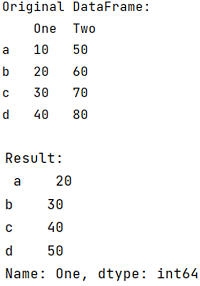# Lambda including if, elif and else

Learn about the Lambda including if, elif and else in Python.
Submitted by Pranit Sharma, on July 07, 2022

Pandas is a special tool that allows us to perform complex manipulations of data effectively and efficiently. Inside pandas, we mostly deal with a dataset in the form of DataFrame. DataFrames are 2-dimensional data structures in pandas. DataFrames consist of rows, columns, and data.

To use the lambda function including if, elif, and else. we need to use the lambda() function.

Lambda function is like a comprehension function inside which any variable can be defined and that variable is also manipulated in the same line using any particular operator.

To work with pandas, we need to import pandas package first, below is the syntax:

```import pandas as pd
```

Let us understand with the help of an example,

## Python code for 'Lambda including if, elif and else'

```# Importing pandas package
import pandas as pd

# Creating a dictionary
d = {
'One':[10,20,30,40],
'Two':[50,60,70,80]
}

# Creating a DataFrame
df = pd.DataFrame(d,index=['a','b','c','d'])

# Display original DataFrame
print("Original DataFrame:\n",df,"\n")

# Applying lambda function
result = df['One'].apply(lambda x: x*10 if x<2 else (x**2 if x<4 else x+10))

# Display result
print("Result:\n",result)
```

Output:What's New (MCQs)

Top Interview Coding Problems/Challenges!

IncludeHelp's Blogs

Languages: » C » C++ » C++ STL » Java » Data Structure » C#.Net » Android » Kotlin » SQL
Web Technologies: » PHP » Python » JavaScript » CSS » Ajax » Node.js » Web programming/HTML
Solved programs: » C » C++ » DS » Java » C#
Aptitude que. & ans.: » C » C++ » Java » DBMS
Interview que. & ans.: » C » Embedded C » Java » SEO » HR
CS Subjects: » CS Basics » O.S. » Networks » DBMS » Embedded Systems » Cloud Computing
» Machine learning » CS Organizations » Linux » DOS
More: » Articles » Puzzles » News/Updates